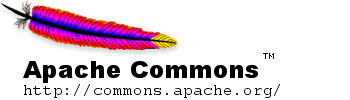## Overview

### 0.1 About The User Guide

This guide is intended to help programmers quickly find what they need to develop solutions using Commons Math. It also provides a supplement to the javadoc API documentation, providing a little more explanation of the mathematical objects and functions included in the package.

### 0.2 What's in commons-math

Commons Math is made up of a small set of math/stat utilities addressing programming problems like the ones in the list below. This list is not exhaustive, it's just meant to give a feel for the kinds of things that Commons Math provides.

• Computing means, variances and other summary statistics for a list of numbers
• Fitting a line to a set of data points using linear regression
• Fitting a curve to a set of data points
• Finding a smooth curve that passes through a collection of points (interpolation)
• Fitting a parametric model to a set of measurements using least-squares methods
• Solving equations involving real-valued functions (i.e. root-finding)
• Solving systems of linear equations
• Solving Ordinary Differential Equations
• Minimizing multi-dimensional functions
• Generating random numbers with more restrictions (e.g distribution, range) than what is possible using the JDK
• Generating random samples and/or datasets that are "like" the data in an input file
• Performing statistical significance tests
• Miscellaneous mathematical functions such as factorials, binomial coefficients and "special functions" (e.g. gamma, beta functions)

We are actively seeking ideas for additional components that fit into the Commons Math vision of a set of lightweight, self-contained math/stat components useful for solving common programming problems. Suggestions for new components or enhancements to existing functionality are always welcome! All feedback/suggestions for improvement should be sent to the commons-dev mailing list with [math] at the beginning of the subject line.

### 0.3 How commons-math is organized

Commons Math is divided into sixteen subpackages, based on functionality provided.

### 0.4 How interface contracts are specified in commons-math javadoc

You should always read the javadoc class and method comments carefully when using Commons Math components in your programs. The javadoc provides references to the algorithms that are used, usage notes about limitations, performance, etc. as well as interface contracts. Interface contracts are specified in terms of preconditions (what has to be true in order for the method to return valid results), special values returned (e.g. Double.NaN) or exceptions that may be thrown if the preconditions are not met, and definitions for returned values/objects or state changes.

When the actual parameters provided to a method or the internal state of an object make a computation meaningless, a MathIllegalArgumentException or MathIllegalStateException may be thrown. Exact conditions under which runtime exceptions (and any other exceptions) are thrown are specified in the javadoc method comments. In some cases, to be consistent with the IEEE 754 standard for floating point arithmetic and with java.lang.Math, Commons Math methods return Double.NaN values. Conditions under which Double.NaN or other special values are returned are fully specified in the javadoc method comments.

As of version 2.2, the policy for dealing with null references is as follows: When an argument is unexpectedly null, a NullArgumentException is raised to signal the illegal argument. Note that this class does not inherit from the standard NullPointerException but is a subclass of MathIllegalArgumentException.

### 0.5 Dependencies

Commons Math requires JDK 1.5+ and has no runtime dependencies.